Скачать презентацию Generating Test Data for Killing SQL Mutants A

646e137fe9668377d9f4926578d36812.ppt

• Количество слайдов: 38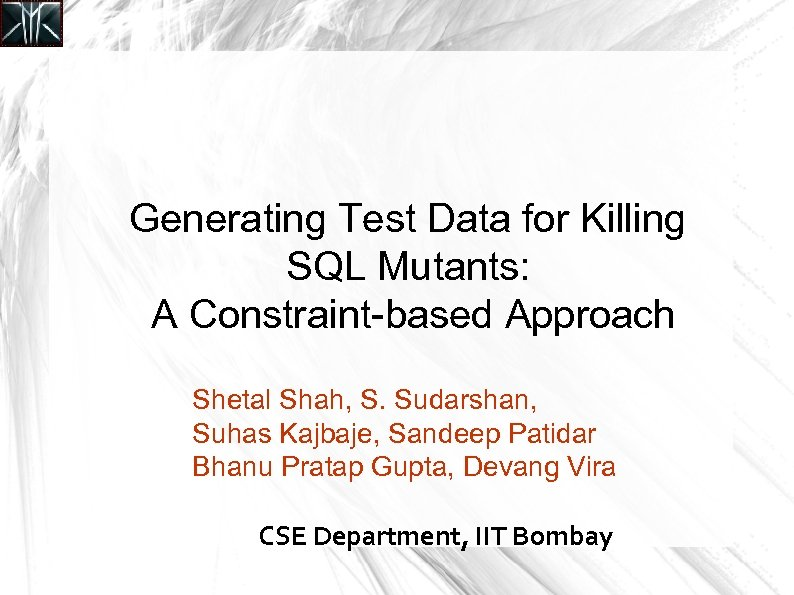Generating Test Data for Killing SQL Mutants: A Constraint-based Approach Shetal Shah, S. Sudarshan, Suhas Kajbaje, Sandeep Patidar Bhanu Pratap Gupta, Devang Vira CSE Department, IIT Bombay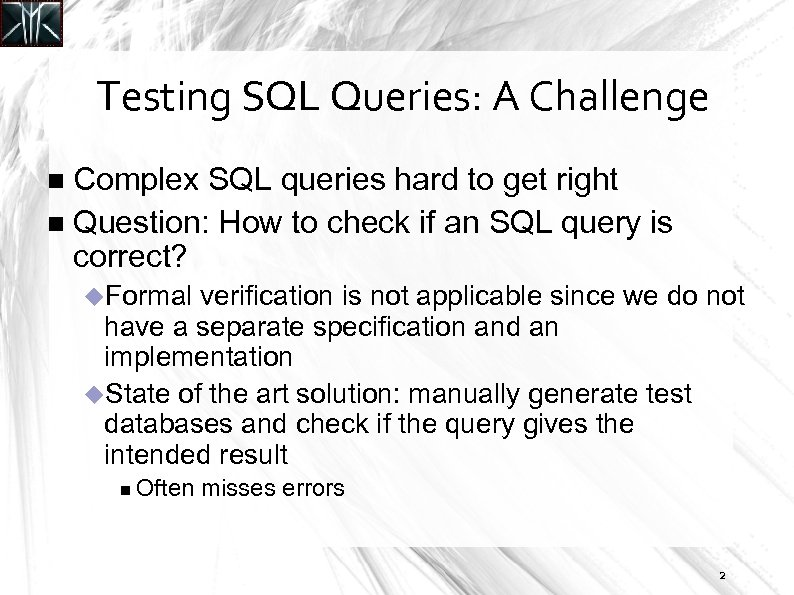Testing SQL Queries: A Challenge Complex SQL queries hard to get right Question: How to check if an SQL query is correct? Formal verification is not applicable since we do not have a separate specification and an implementation State of the art solution: manually generate test databases and check if the query gives the intended result Often misses errors 2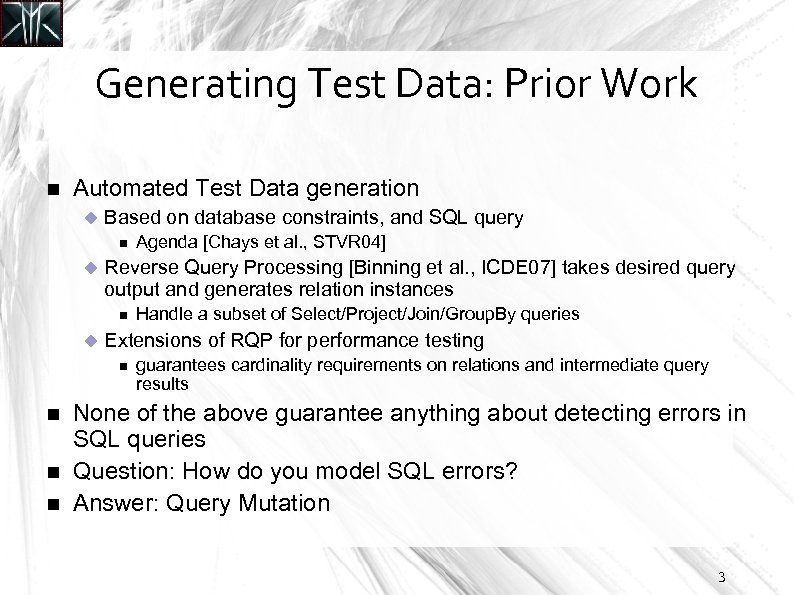Generating Test Data: Prior Work Automated Test Data generation Based on database constraints, and SQL query Reverse Query Processing [Binning et al. , ICDE 07] takes desired query output and generates relation instances Handle a subset of Select/Project/Join/Group. By queries Extensions of RQP for performance testing Agenda [Chays et al. , STVR 04] guarantees cardinality requirements on relations and intermediate query results None of the above guarantee anything about detecting errors in SQL queries Question: How do you model SQL errors? Answer: Query Mutation 3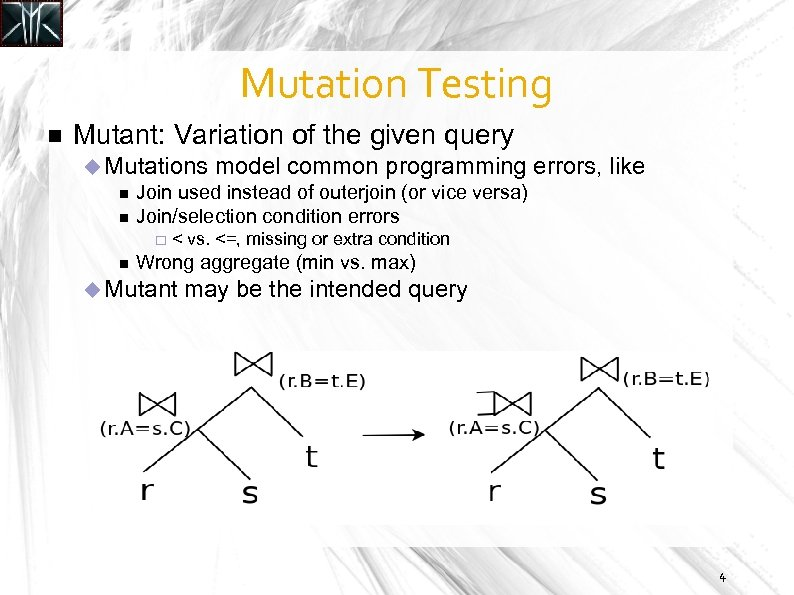Mutation Testing Mutant: Variation of the given query Mutations model common programming errors, Join used instead of outerjoin (or vice versa) Join/selection condition errors like < vs. <=, missing or extra condition Wrong aggregate (min vs. max) Mutant may be the intended query 4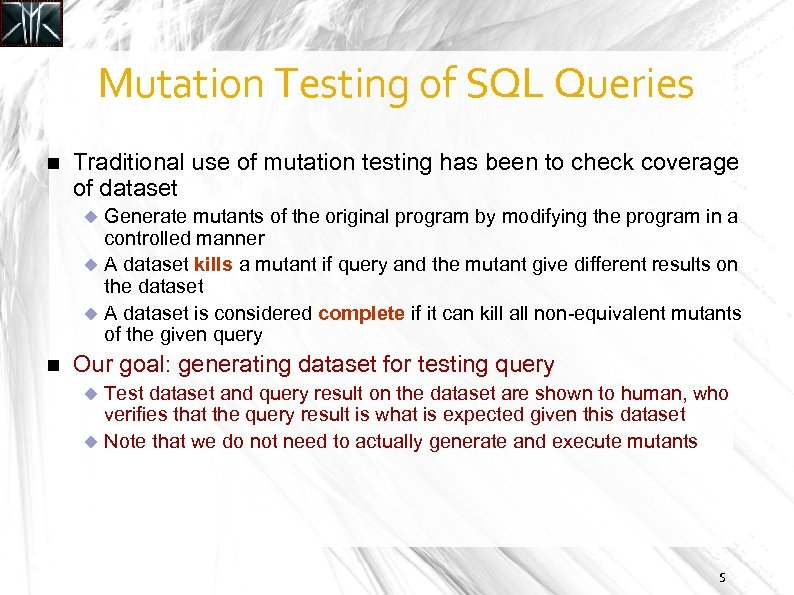Mutation Testing of SQL Queries Traditional use of mutation testing has been to check coverage of dataset Generate mutants of the original program by modifying the program in a controlled manner A dataset kills a mutant if query and the mutant give different results on the dataset A dataset is considered complete if it can kill all non-equivalent mutants of the given query Our goal: generating dataset for testing query Test dataset and query result on the dataset are shown to human, who verifies that the query result is what is expected given this dataset Note that we do not need to actually generate and execute mutants 5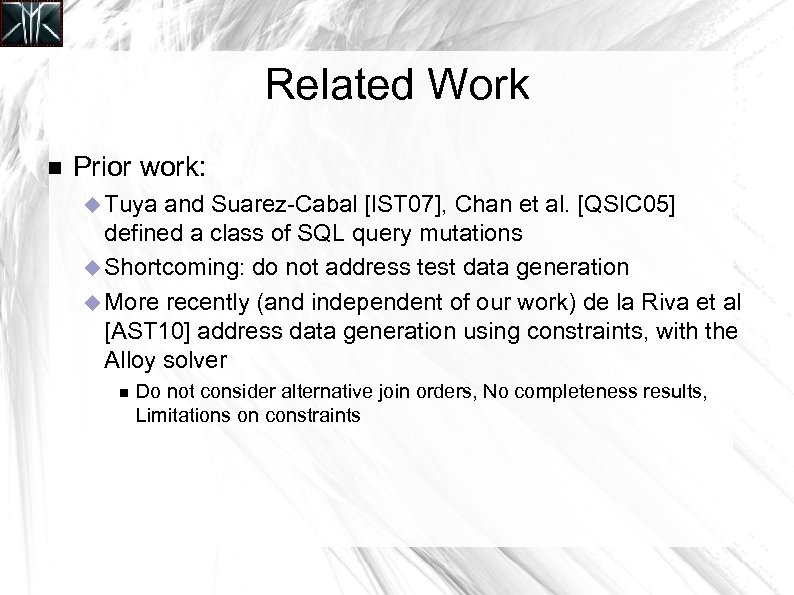Related Work Prior work: Tuya and Suarez-Cabal [IST 07], Chan et al. [QSIC 05] defined a class of SQL query mutations Shortcoming: do not address test data generation More recently (and independent of our work) de la Riva et al [AST 10] address data generation using constraints, with the Alloy solver Do not consider alternative join orders, No completeness results, Limitations on constraints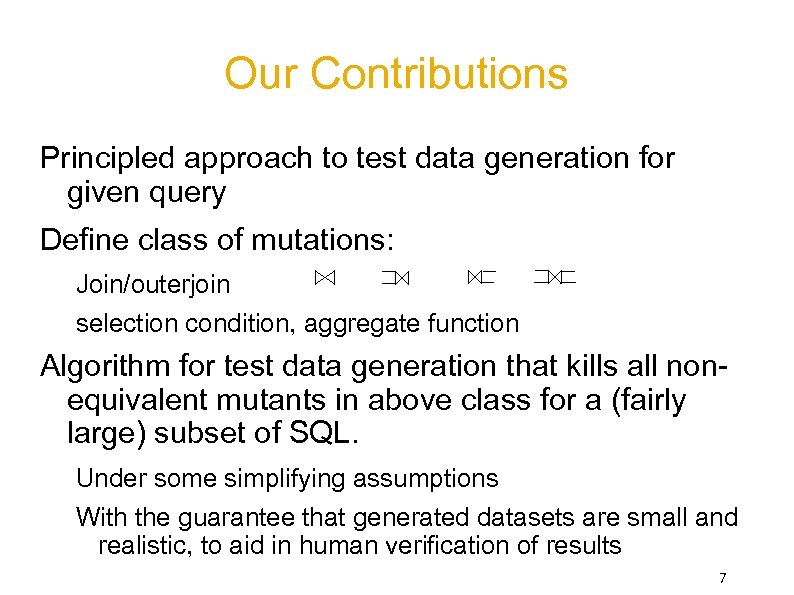Our Contributions Principled approach to test data generation for given query Define class of mutations: Join/outerjoin selection condition, aggregate function Algorithm for test data generation that kills all nonequivalent mutants in above class for a (fairly large) subset of SQL. Under some simplifying assumptions With the guarantee that generated datasets are small and realistic, to aid in human verification of results 7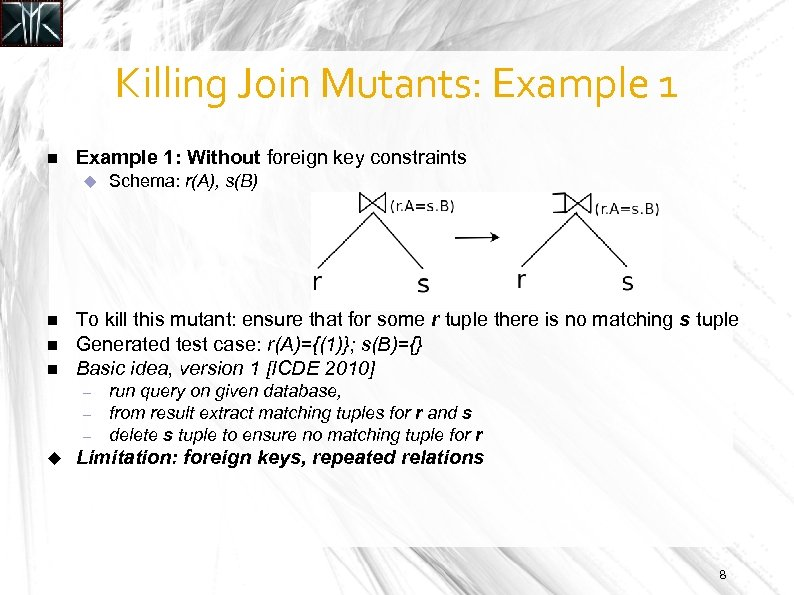Killing Join Mutants: Example 1: Without foreign key constraints To kill this mutant: ensure that for some r tuple there is no matching s tuple Generated test case: r(A)={(1)}; s(B)={} Basic idea, version 1 [ICDE 2010] – – – Schema: r(A), s(B) run query on given database, from result extract matching tuples for r and s delete s tuple to ensure no matching tuple for r Limitation: foreign keys, repeated relations 8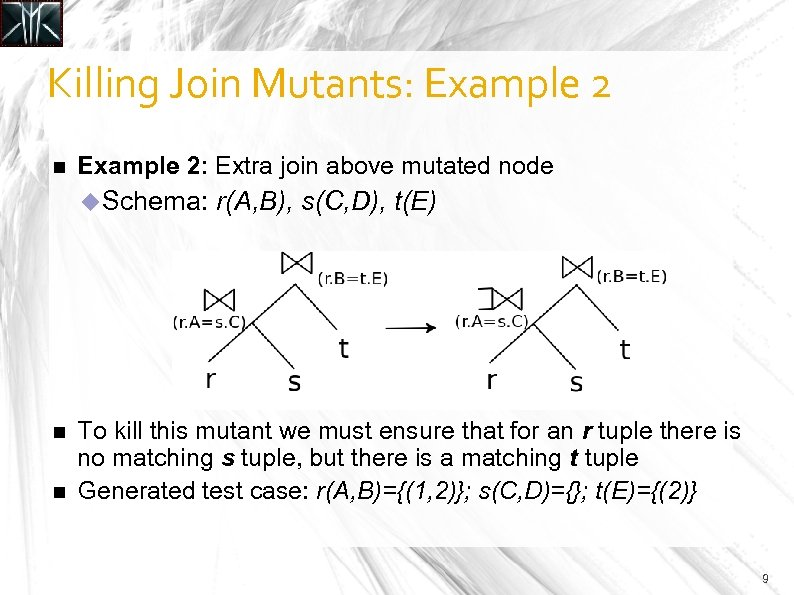Killing Join Mutants: Example 2: Extra join above mutated node Schema: r(A, B), s(C, D), t(E) To kill this mutant we must ensure that for an r tuple there is no matching s tuple, but there is a matching t tuple Generated test case: r(A, B)={(1, 2)}; s(C, D)={}; t(E)={(2)} 9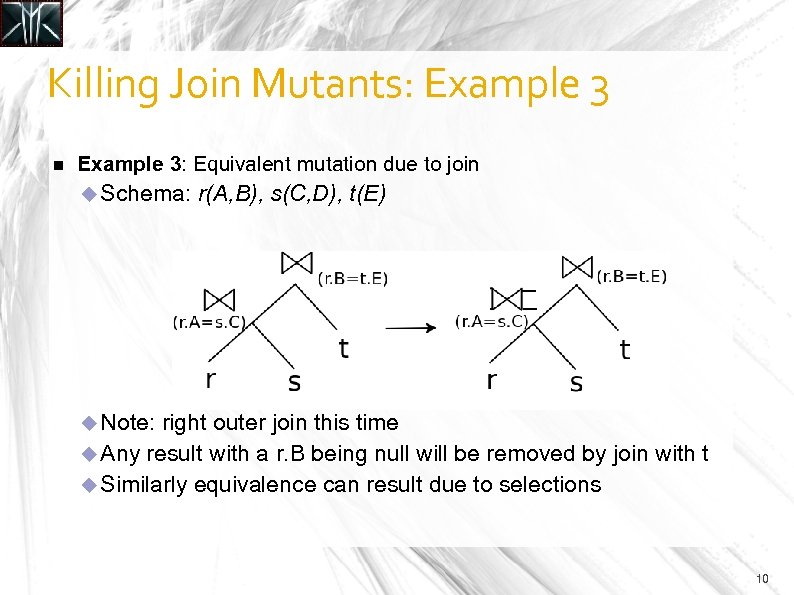Killing Join Mutants: Example 3: Equivalent mutation due to join Schema: r(A, B), s(C, D), t(E) Note: right outer join this time Any result with a r. B being null will be removed by join with t Similarly equivalence can result due to selections 10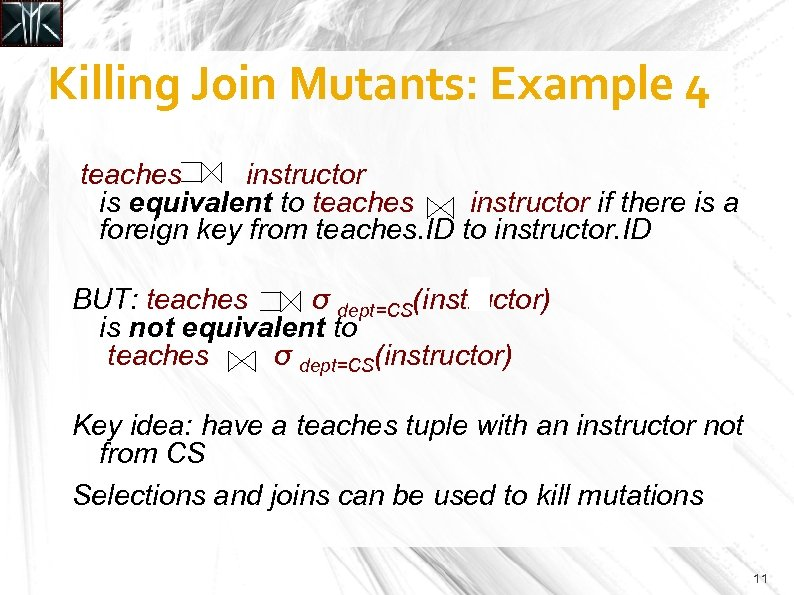Killing Join Mutants: Example 4 teaches instructor is equivalent to teaches instructor if there is a foreign key from teaches. ID to instructor. ID BUT: teaches σ dept=CS(instructor) is not equivalent to teaches σ dept=CS(instructor) Key idea: have a teaches tuple with an instructor not from CS Selections and joins can be used to kill mutations 11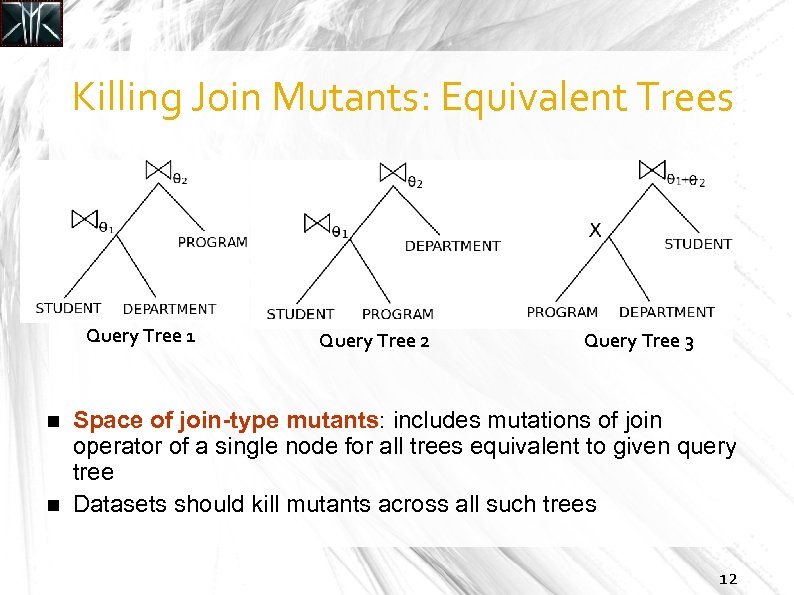Killing Join Mutants: Equivalent Trees Query Tree 1 Query Tree 2 Query Tree 3 Space of join-type mutants: includes mutations of join operator of a single node for all trees equivalent to given query tree Datasets should kill mutants across all such trees 12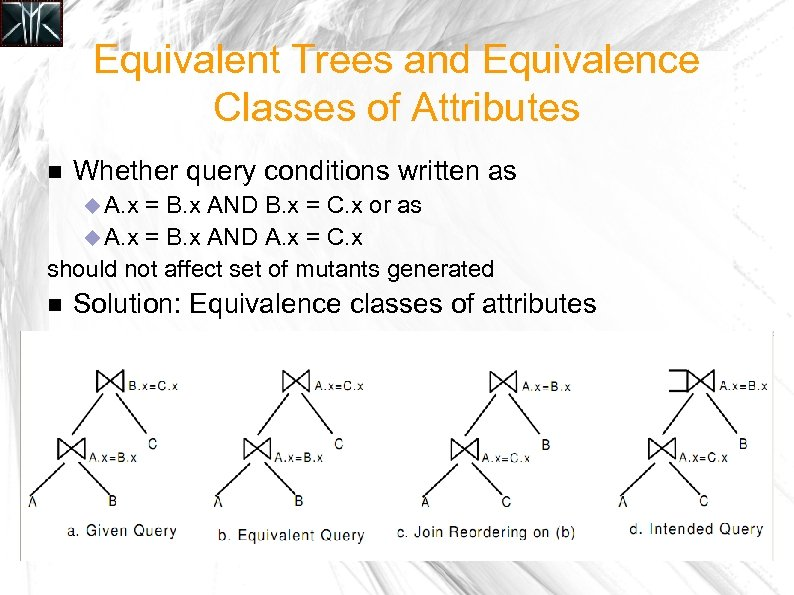Equivalent Trees and Equivalence Classes of Attributes Whether query conditions written as A. x = B. x AND B. x = C. x or as A. x = B. x AND A. x = C. x should not affect set of mutants generated Solution: Equivalence classes of attributes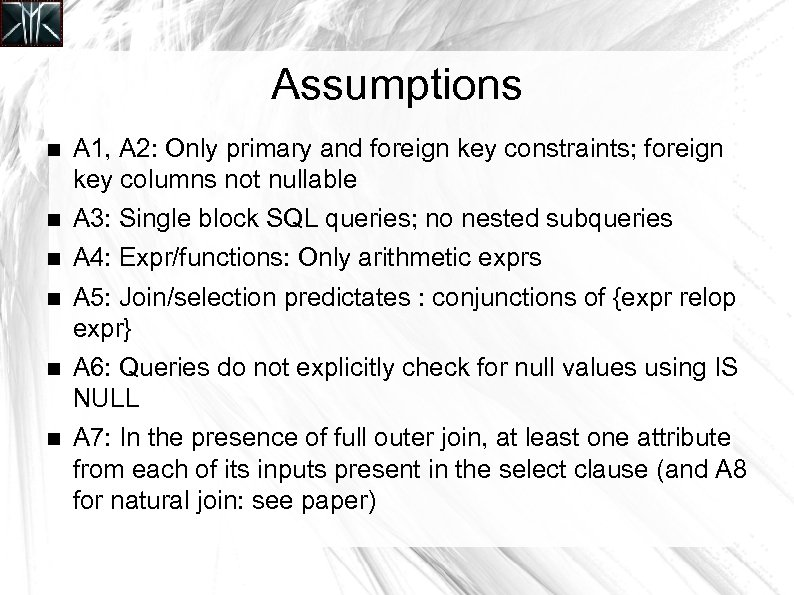Assumptions A 1, A 2: Only primary and foreign key constraints; foreign key columns not nullable A 3: Single block SQL queries; no nested subqueries A 4: Expr/functions: Only arithmetic exprs A 5: Join/selection predictates : conjunctions of {expr relop expr} A 6: Queries do not explicitly check for null values using IS NULL A 7: In the presence of full outer join, at least one attribute from each of its inputs present in the select clause (and A 8 for natural join: see paper)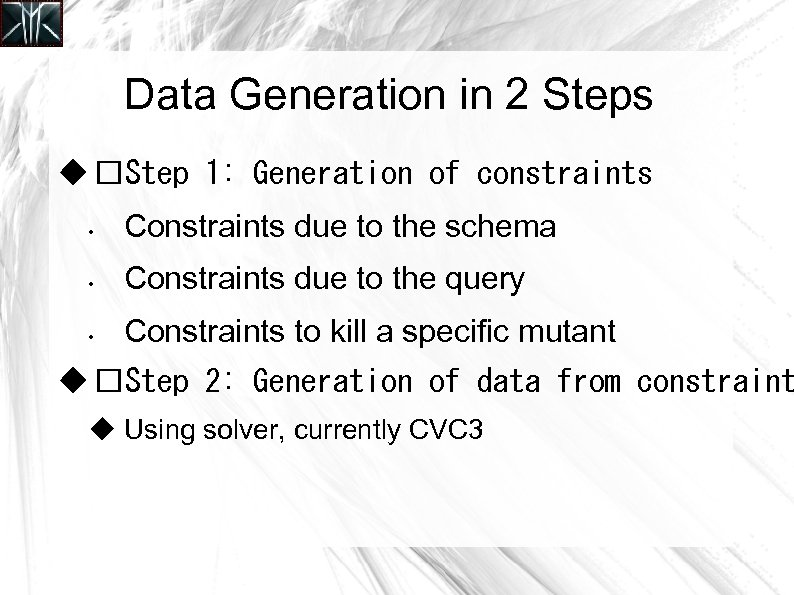Data Generation in 2 Steps Step 1: Generation of constraints • Constraints due to the schema • Constraints due to the query • Constraints to kill a specific mutant Step 2: Generation of data from constraint Using solver, currently CVC 3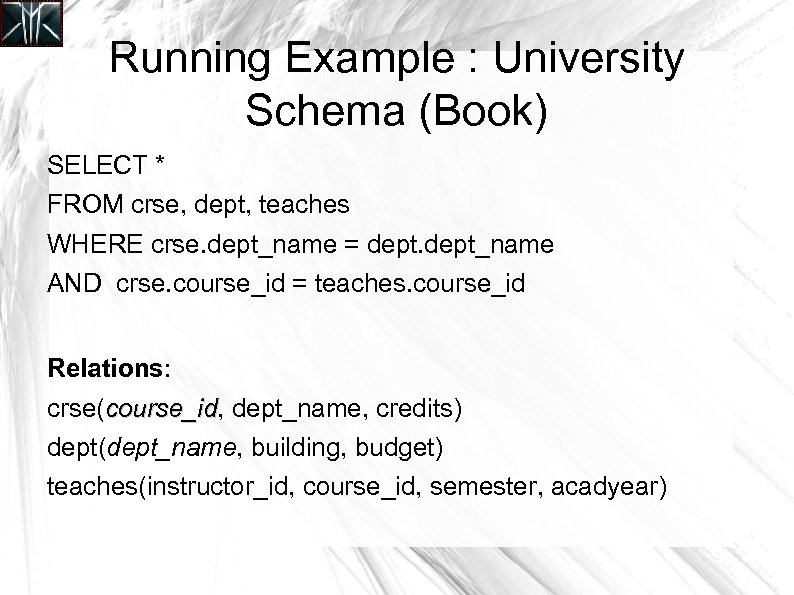Running Example : University Schema (Book) SELECT * FROM crse, dept, teaches WHERE crse. dept_name = dept_name AND crse. course_id = teaches. course_id Relations: crse(course_id, dept_name, credits) course_id dept(dept_name, building, budget) teaches(instructor_id, course_id, semester, acadyear)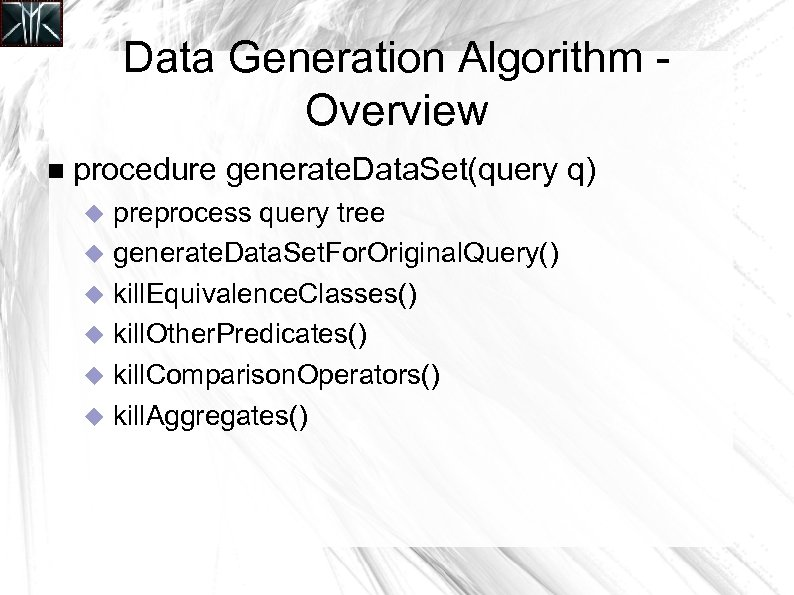Data Generation Algorithm Overview procedure generate. Data. Set(query q) preprocess query tree generate. Data. Set. For. Original. Query() kill. Equivalence. Classes() kill. Other. Predicates() kill. Comparison. Operators() kill. Aggregates()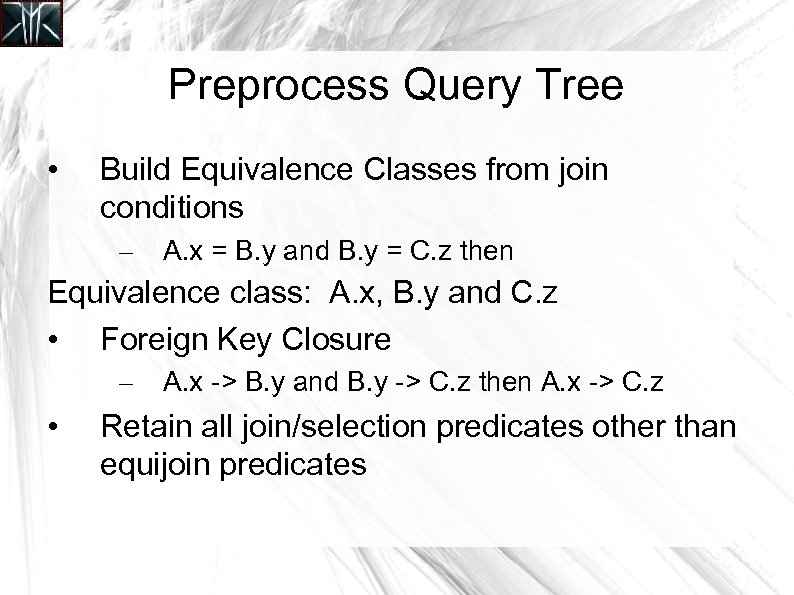Preprocess Query Tree • Build Equivalence Classes from join conditions – A. x = B. y and B. y = C. z then Equivalence class: A. x, B. y and C. z • Foreign Key Closure – • A. x -> B. y and B. y -> C. z then A. x -> C. z Retain all join/selection predicates other than equijoin predicates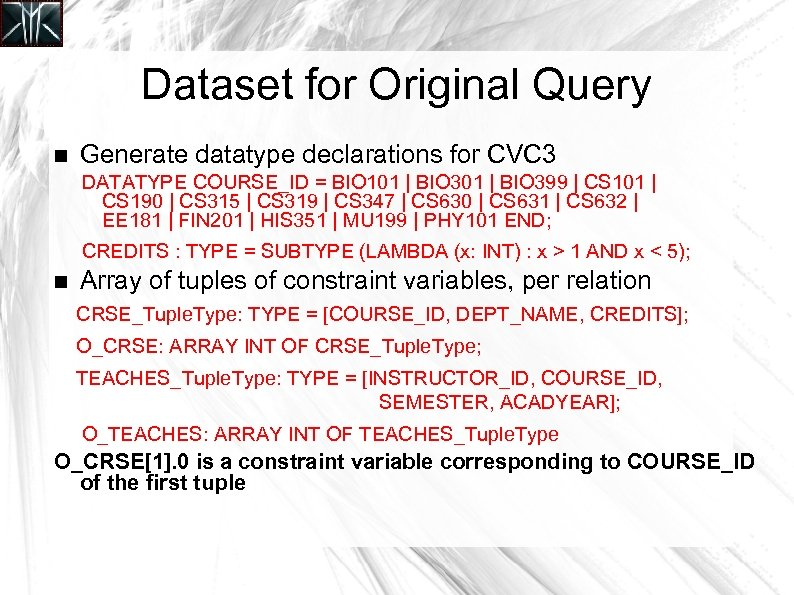Dataset for Original Query Generate datatype declarations for CVC 3 DATATYPE COURSE_ID = BIO 101 | BIO 399 | CS 101 | CS 190 | CS 315 | CS 319 | CS 347 | CS 630 | CS 631 | CS 632 | EE 181 | FIN 201 | HIS 351 | MU 199 | PHY 101 END; CREDITS : TYPE = SUBTYPE (LAMBDA (x: INT) : x > 1 AND x < 5); Array of tuples of constraint variables, per relation CRSE_Tuple. Type: TYPE = [COURSE_ID, DEPT_NAME, CREDITS]; O_CRSE: ARRAY INT OF CRSE_Tuple. Type; TEACHES_Tuple. Type: TYPE = [INSTRUCTOR_ID, COURSE_ID, SEMESTER, ACADYEAR]; O_TEACHES: ARRAY INT OF TEACHES_Tuple. Type O_CRSE. 0 is a constraint variable corresponding to COURSE_ID of the first tuple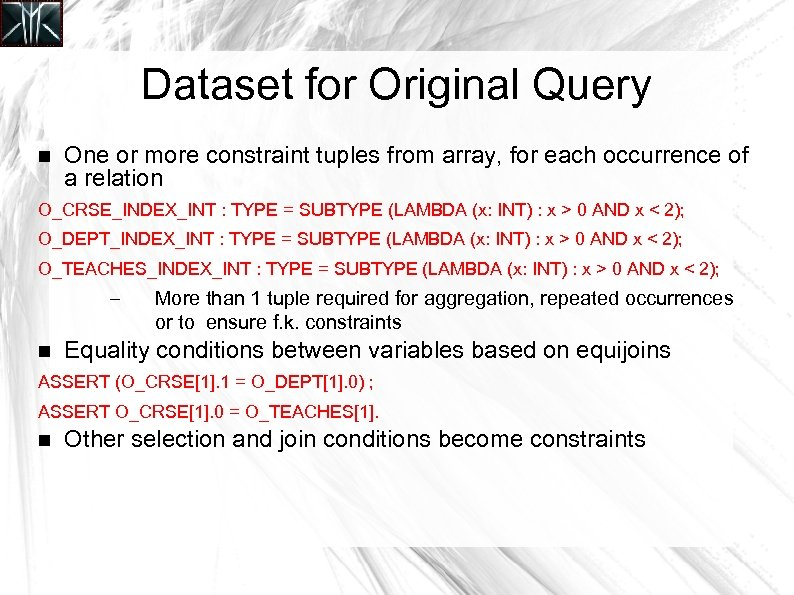Dataset for Original Query One or more constraint tuples from array, for each occurrence of a relation O_CRSE_INDEX_INT : TYPE = SUBTYPE (LAMBDA (x: INT) : x > 0 AND x < 2); O_DEPT_INDEX_INT : TYPE = SUBTYPE (LAMBDA (x: INT) : x > 0 AND x < 2); O_TEACHES_INDEX_INT : TYPE = SUBTYPE (LAMBDA (x: INT) : x > 0 AND x < 2); – More than 1 tuple required for aggregation, repeated occurrences or to ensure f. k. constraints Equality conditions between variables based on equijoins ASSERT (O_CRSE. 1 = O_DEPT. 0) ; ASSERT O_CRSE. 0 = O_TEACHES. Other selection and join conditions become constraints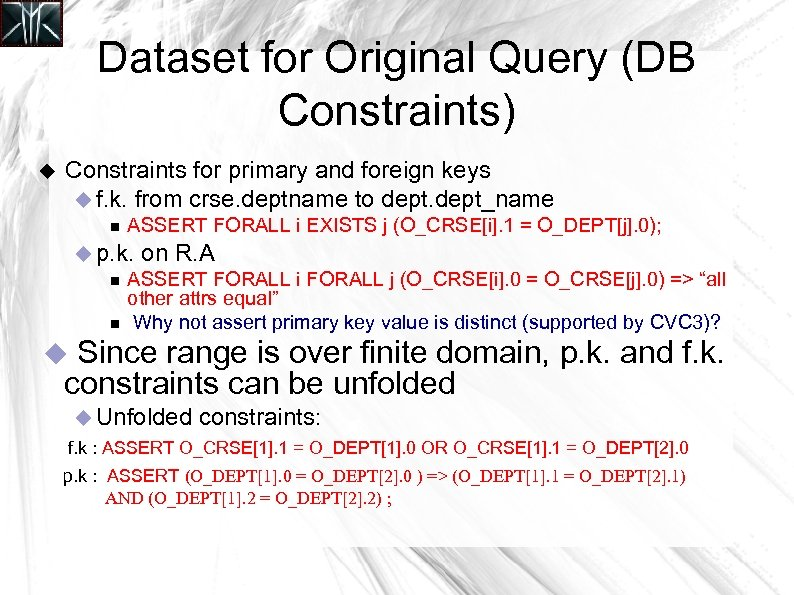Dataset for Original Query (DB Constraints) Constraints for primary and foreign keys f. k. from crse. deptname to dept_name ASSERT FORALL i EXISTS j (O_CRSE[i]. 1 = O_DEPT[j]. 0); p. k. on R. A ASSERT FORALL i FORALL j (O_CRSE[i]. 0 = O_CRSE[j]. 0) => “all other attrs equal” Why not assert primary key value is distinct (supported by CVC 3)? Since range is over finite domain, p. k. and f. k. constraints can be unfolded Unfolded constraints: f. k : ASSERT O_CRSE. 1 = O_DEPT. 0 OR O_CRSE. 1 = O_DEPT. 0 p. k : ASSERT (O_DEPT. 0 = O_DEPT. 0 ) => (O_DEPT. 1 = O_DEPT. 1) AND (O_DEPT. 2 = O_DEPT. 2) ;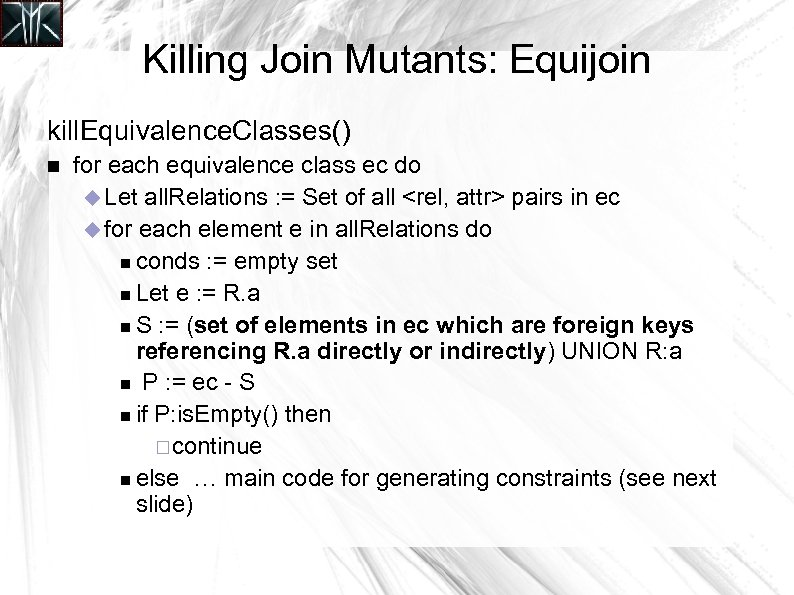Killing Join Mutants: Equijoin kill. Equivalence. Classes() for each equivalence class ec do Let all. Relations : = Set of all pairs in ec for each element e in all. Relations do conds : = empty set Let e : = R. a S : = (set of elements in ec which are foreign keys referencing R. a directly or indirectly) UNION R: a P : = ec - S if P: is. Empty() then continue else … main code for generating constraints (see next slide)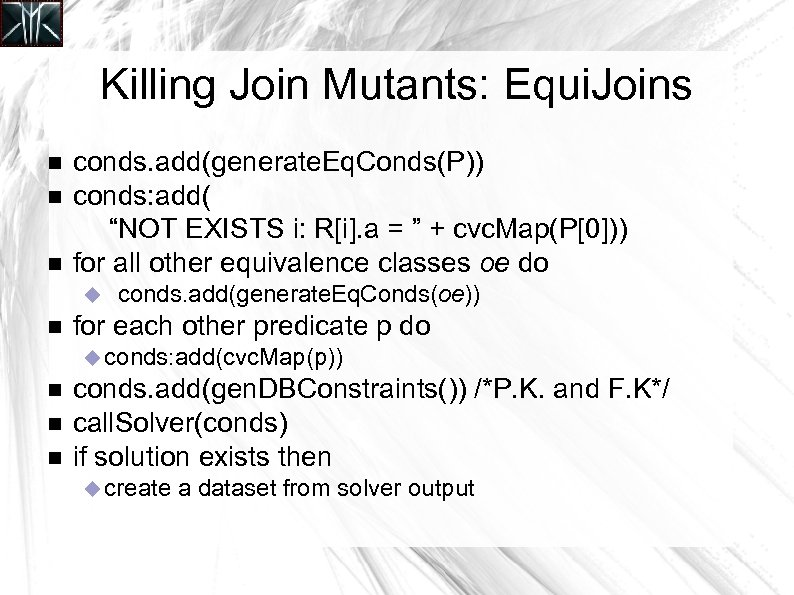Killing Join Mutants: Equi. Joins conds. add(generate. Eq. Conds(P)) conds: add( “NOT EXISTS i: R[i]. a = ” + cvc. Map(P)) for all other equivalence classes oe do conds. add(generate. Eq. Conds(oe)) for each other predicate p do conds: add(cvc. Map(p)) conds. add(gen. DBConstraints()) /*P. K. and F. K*/ call. Solver(conds) if solution exists then create a dataset from solver output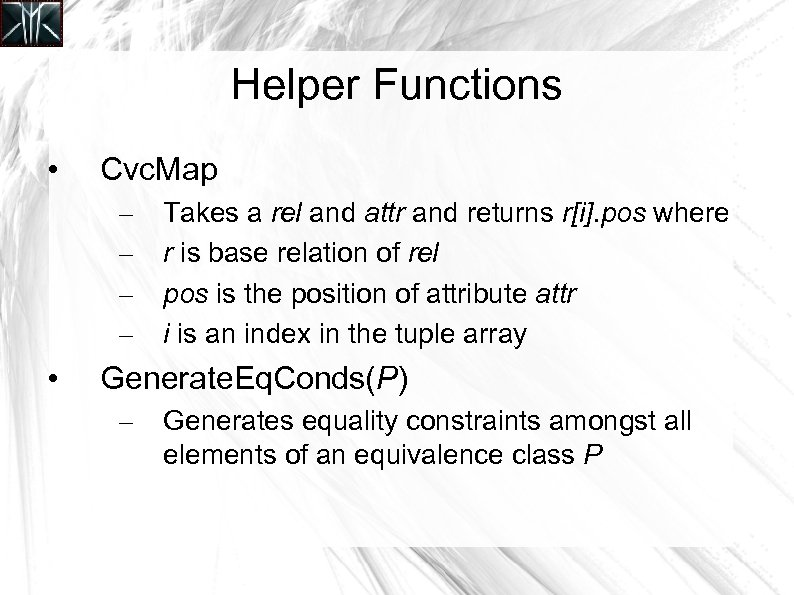Helper Functions • Cvc. Map – – • Takes a rel and attr and returns r[i]. pos where r is base relation of rel pos is the position of attribute attr i is an index in the tuple array Generate. Eq. Conds(P) – Generates equality constraints amongst all elements of an equivalence class P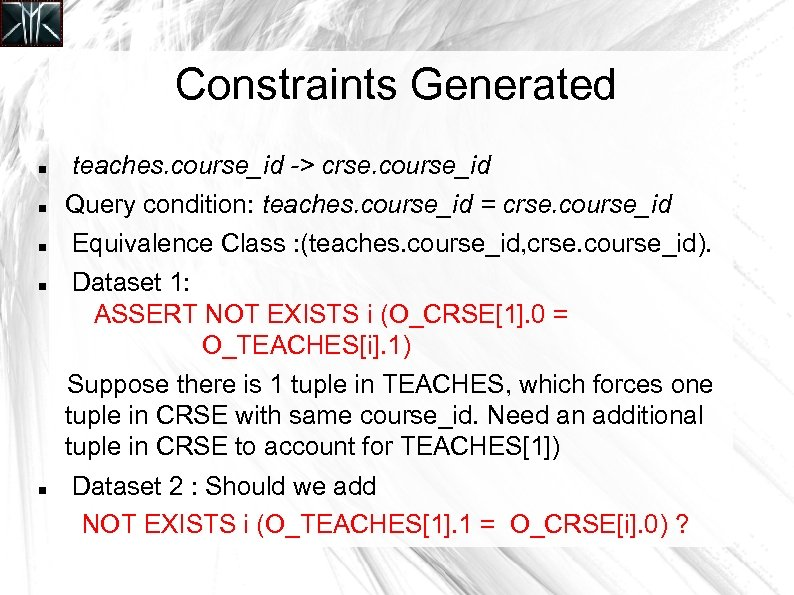Constraints Generated teaches. course_id -> crse. course_id Query condition: teaches. course_id = crse. course_id Equivalence Class : (teaches. course_id, crse. course_id). Dataset 1: ASSERT NOT EXISTS i (O_CRSE. 0 = O_TEACHES[i]. 1) Suppose there is 1 tuple in TEACHES, which forces one tuple in CRSE with same course_id. Need an additional tuple in CRSE to account for TEACHES) Dataset 2 : Should we add NOT EXISTS i (O_TEACHES. 1 = O_CRSE[i]. 0) ?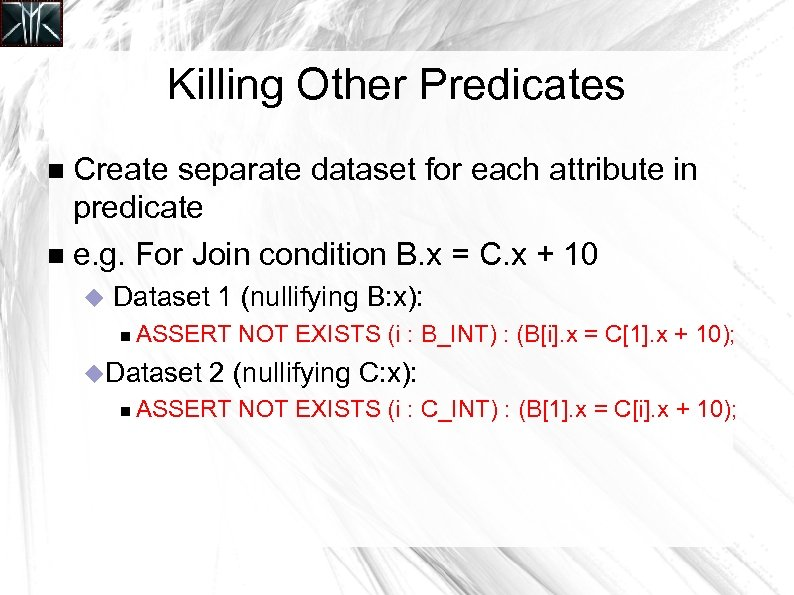Killing Other Predicates Create separate dataset for each attribute in predicate e. g. For Join condition B. x = C. x + 10 Dataset 1 (nullifying B: x): ASSERT NOT EXISTS (i : B_INT) : (B[i]. x = C. x + 10); Dataset 2 (nullifying C: x): ASSERT NOT EXISTS (i : C_INT) : (B. x = C[i]. x + 10);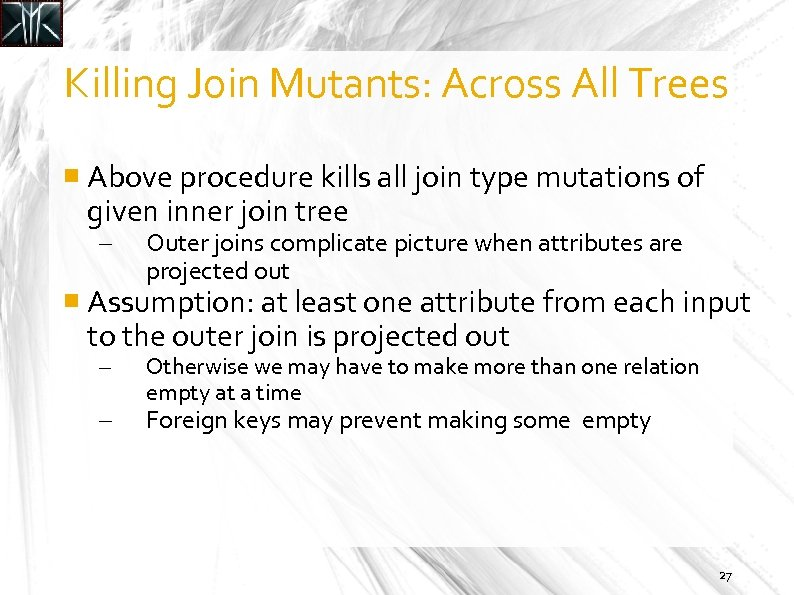Killing Join Mutants: Across All Trees Above procedure kills all join type mutations of given inner join tree – Outer joins complicate picture when attributes are projected out Assumption: at least one attribute from each input to the outer join is projected out – – Otherwise we may have to make more than one relation empty at a time Foreign keys may prevent making some empty 27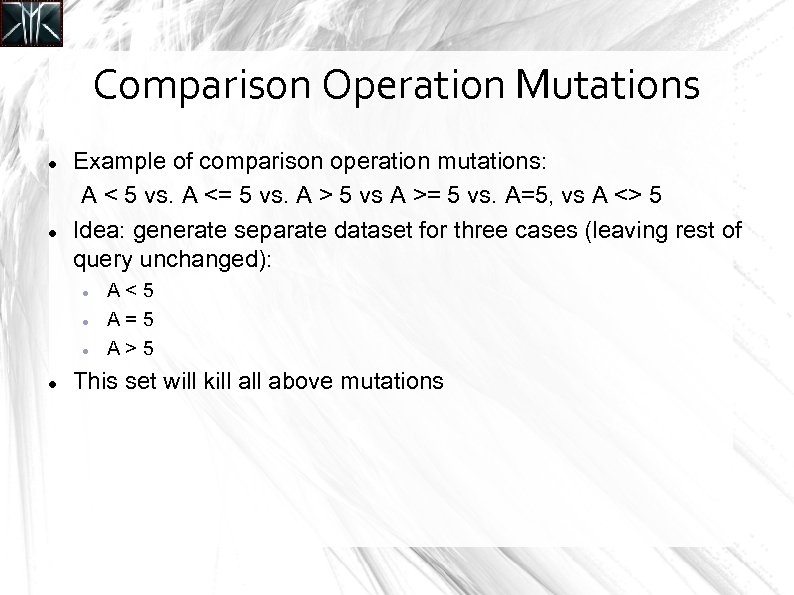Comparison Operation Mutations Example of comparison operation mutations: A < 5 vs. A <= 5 vs. A > 5 vs A >= 5 vs. A=5, vs A <> 5 Idea: generate separate dataset for three cases (leaving rest of query unchanged): A<5 A=5 A>5 This set will kill above mutations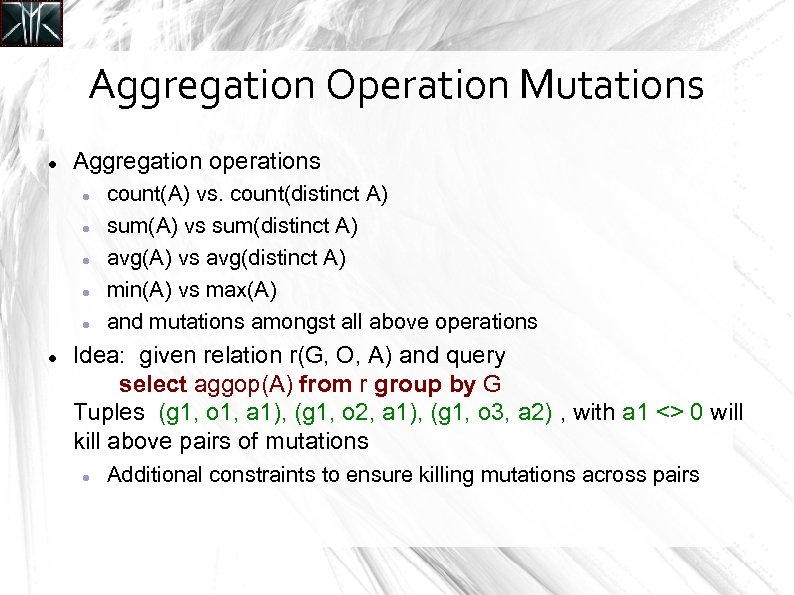Aggregation Operation Mutations Aggregation operations count(A) vs. count(distinct A) sum(A) vs sum(distinct A) avg(A) vs avg(distinct A) min(A) vs max(A) and mutations amongst all above operations Idea: given relation r(G, O, A) and query select aggop(A) from r group by G Tuples (g 1, o 1, a 1), (g 1, o 2, a 1), (g 1, o 3, a 2) , with a 1 <> 0 will kill above pairs of mutations Additional constraints to ensure killing mutations across pairs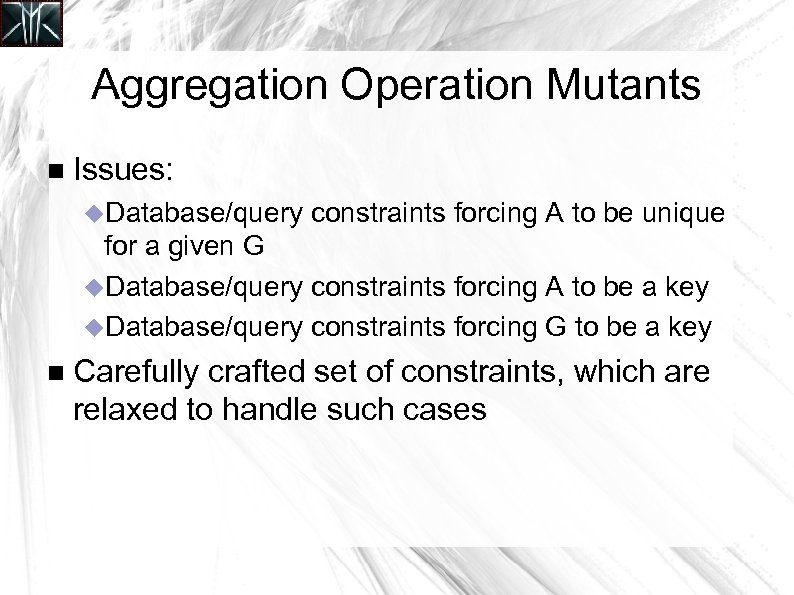Aggregation Operation Mutants Issues: Database/query constraints forcing A to be unique for a given G Database/query constraints forcing A to be a key Database/query constraints forcing G to be a key Carefully crafted set of constraints, which are relaxed to handle such cases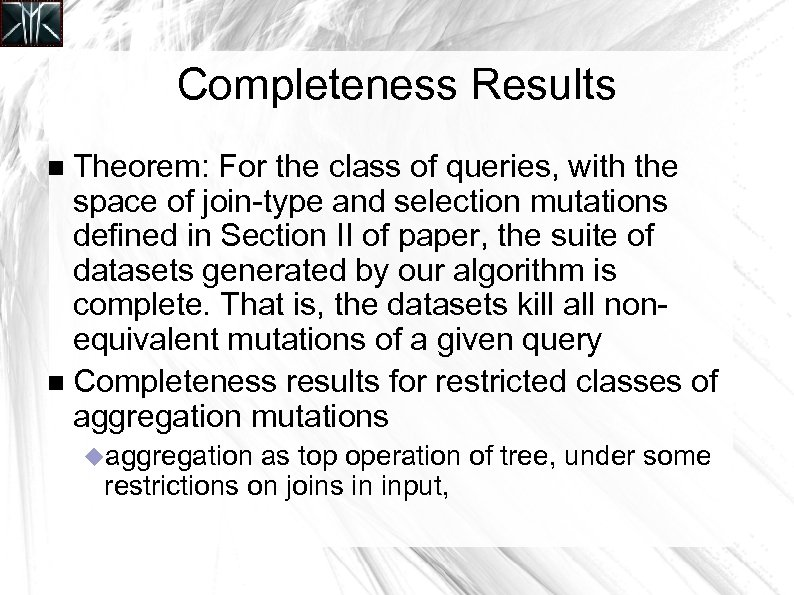Completeness Results Theorem: For the class of queries, with the space of join-type and selection mutations deﬁned in Section II of paper, the suite of datasets generated by our algorithm is complete. That is, the datasets kill all nonequivalent mutations of a given query Completeness results for restricted classes of aggregation mutations aggregation as top operation of tree, under some restrictions on joins in input,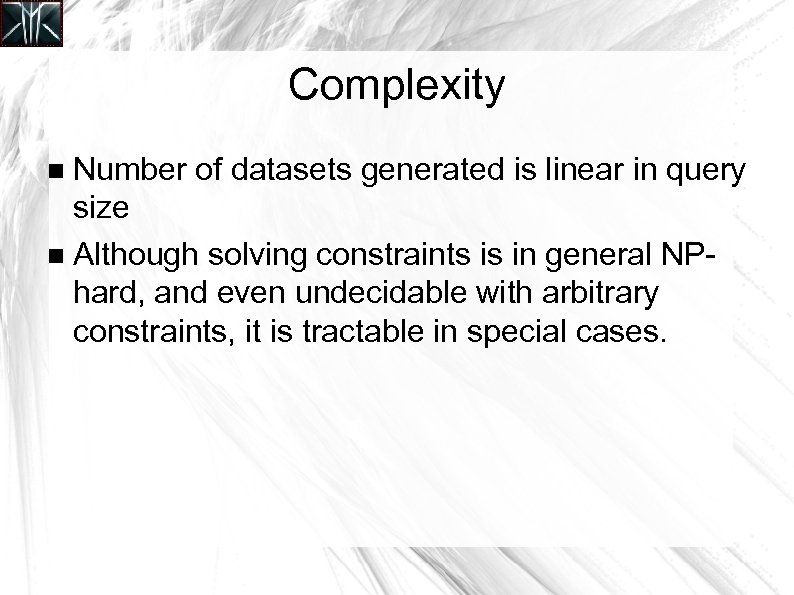Complexity Number of datasets generated is linear in query size Although solving constraints is in general NPhard, and even undecidable with arbitrary constraints, it is tractable in special cases.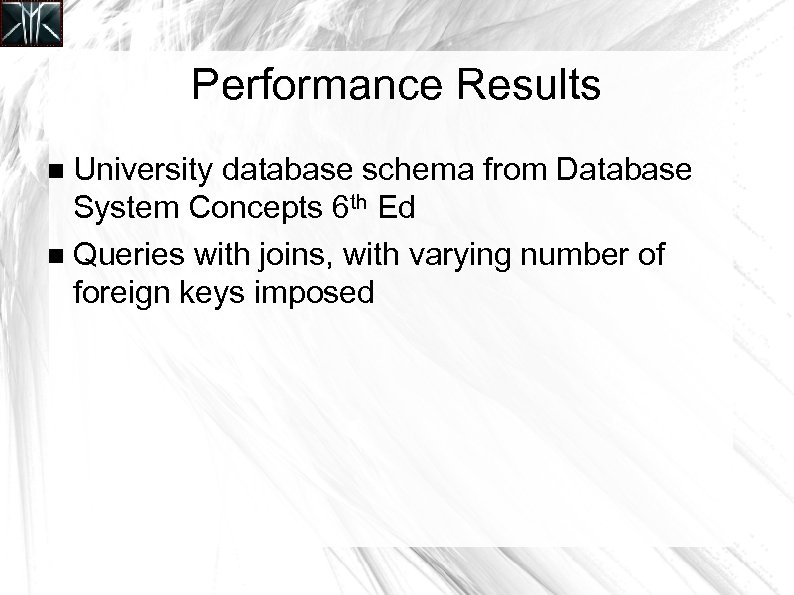Performance Results University database schema from Database System Concepts 6 th Ed Queries with joins, with varying number of foreign keys imposed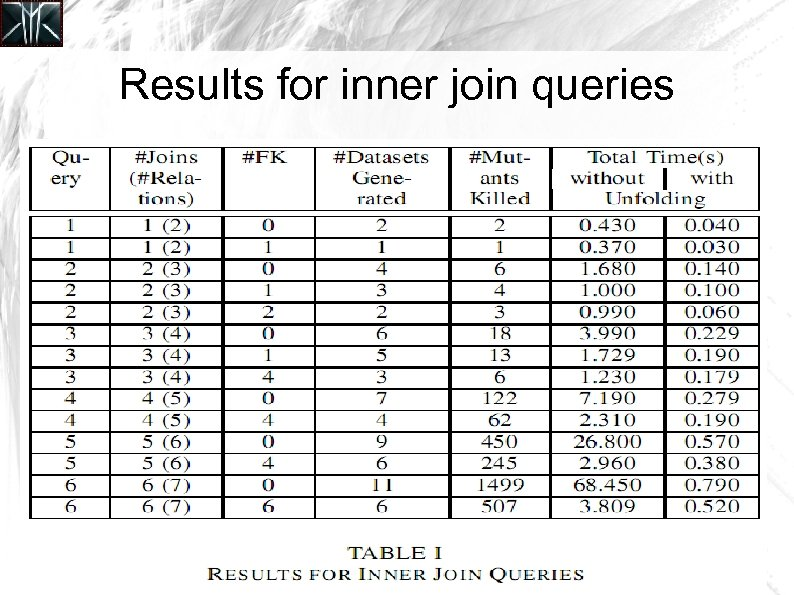Results for inner join queries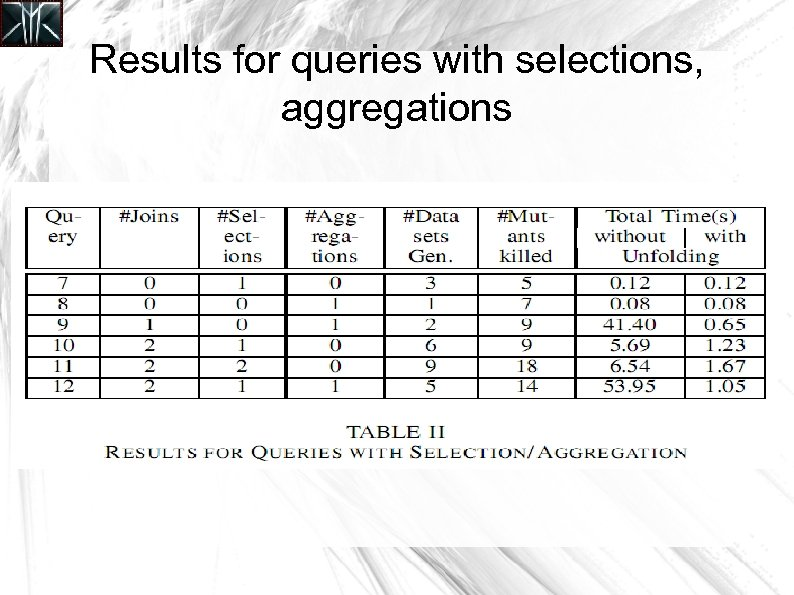Results for queries with selections, aggregationsOngoing and Future Work Ongoing work Handling aggregations and sub-queries Other mutations missing/extra join conditions missing/extra selection conditions E. g. student. Active = 'Y' Future work: data generation for applications multiple queries form parameters 36Questions Thank You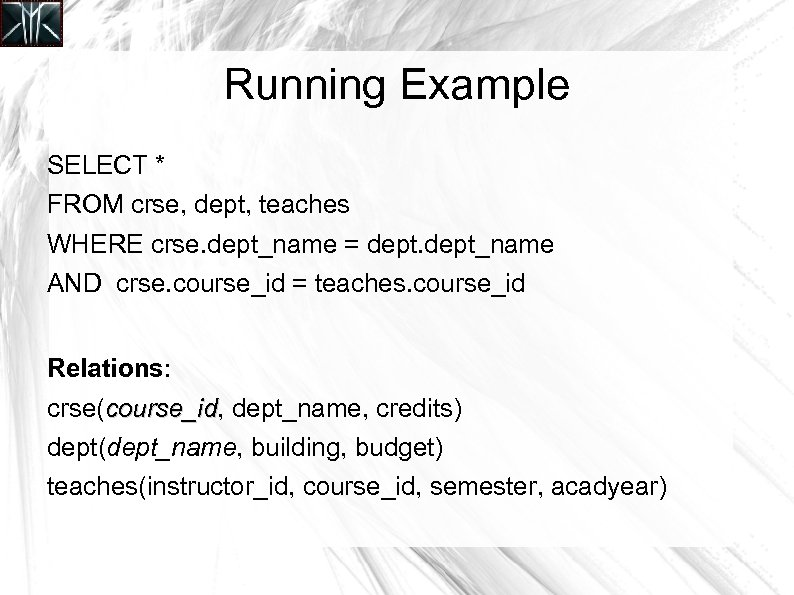Running Example SELECT * FROM crse, dept, teaches WHERE crse. dept_name = dept_name AND crse. course_id = teaches. course_id Relations: crse(course_id, dept_name, credits) course_id dept(dept_name, building, budget) teaches(instructor_id, course_id, semester, acadyear)